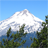# ArcGIS Pattern and Cluster Analysis Tools

873
3
06-06-2012 02:39 AMNew Contributor
I want to categorize all the landmines in a minefield into one cluster or another.  I do not want to use anything related to statistical significance for doing so, e.g. z-scores, p-values.  Rather, it is more of a classification problem than a hotspot analysis.  Let me try to explain:

Using a 1-dimensional analogue, consider:

x  x    xx   x      xxxxx   xxx

If we need at least two x's to be in any given cluster,  the above might be considered as 4 clusters (sizes 2,3,5,3 going left to right) or three clusters (5,5,3) or 4 clusters with an outlier (2,2,outlier, 5,3).  An algorithm might try putting these together in various ways, each time calculating the ratio of between-cluster variance to within-cluster distance variance, and choose the categorization that
maximizes this ratio.
Does anybody knows if ArcGIS has a procedure that could figure out for a given minefield what are the best categorizations that result in 2,3, or 4 clusters, then choose the number (2,3,or 4) that is 'best' in some sense, then labels each mine as to being in which cluster?
Tags (3)
3 RepliesbyEsri Regular Contributor
If these are point features, you can try the following:

1. Run the Buffer tool with a distance that is big enough so that clustered points are enclosed (grouped) by the buffer polygons.
2. Run the Spatial Join tool with JOIN_ONE_TO_ONE option to join the buffers that CONTAINS points. The output should have a JOIN_COUNT field with the count numbers.
3. Run the Intersect tool to intersect the points with the output step 2. Now the points in one cluster should have the same unique buffer polygon ID (classification) and the count.

All three tools are in Analysis toolbox - Proximity or Overlay toolset.

Does that work for you?New Contributor
Hi Dan,

Thanks for the info. This does help in the sense that I find the mines within the buffer however I do not want the specific mine (point) to be in more than one cluster. I was hoping that maybe with some sort of statistical method I can identify the clusters.

Thanks
HansiebyEsri Regular Contributor
Hi Hansie,

If a point satisfies the same criteria that defines a cluster (in this case the buffere distance) and falls in multiple clusters, you can find them using Spatial Join tool to join the points with the buffers with the JOIN_ONE_TO_ONE option. The output contains a JOIN_COUNT field; any value > 1 in this field indicates that the point is in that number of clusters/buffers).

You would need additional criteria to further determine which cluster such point should belong to. What's your thoughts on that? Can you describe or show examples how you wish a point to be included in one cluster, not another?